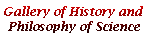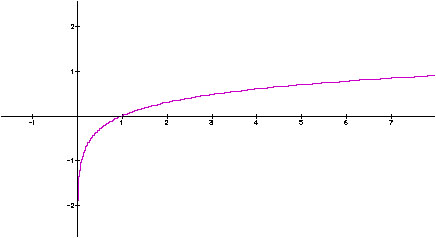Å@

Daniel Bernoulli and the St. Petersburg Paradox

The Bernoulli family is famous for a number of distinguished mathematicians. Daniel is a son of Johann (Jean) I, who was a younger brother of Jakob (Jacques), the author of Ars Conjectandi. Despite Johann's objections Daniel became a mathematician himself, and Daniel spent several years in St. Petersburg, as a professor of mathematics. This period seems to be most fruitful for Daniel, partly because of his collaboration with Leonhard Euler. Daniel produced significant results in hydrodynamics, probability, and became a precursor of the kinetic theory of gases.

However, Daniel did not like the climate of Russia and went back to Basel (Switzerland) and taught there. But, since he shared with his father Johann the Grand Prix of Paris Academy for 1734, the father became angry, and their relationships were seriously damaged.

In his paper on probability and expectation, published in 1738 (but written much earlier) by St. Petersburg Academy, he discussed a paradox now known as the "St. Petersburg Paradox". Suppose a fair coin is tossed, and you will receive \2 if it shows head for the first time on the first trial, \4 if it shows head for the first time on the second trial, \8 if head appears for the first time on the third trial, and so on. The money is doubled each time in this way. Now what is the fair price for joining this game? Or, how much are you ready to pay for this game?

According to the formula of mathematical expectation, the expected value of this game is

(1/2)2 + (1/4)4 + (1/8)8 + . . .

so that, as you can easily see, the expected value is infinite! However, no one is willing to pay even a modest amount of money, say \100, for this game. Then, is the notion of mathematical expectation wrong? Whence this gap between mathematics and our intuitions? This is the St. Petersburg Paradox.

Daniel thought that the value of any amout of money is determined relative to one's whole wealth, and its contribution to the value of the whole is inversely proportional to the whole. In symbols, letting y the value (now called utility) and x the amount of money,

dy = kdx/x (k is a constant).

This makes y a logarithmic functin of x,

y =k log x + constant

so that the value of the same amount of money decreases as your wealth becomes larger. This property is a form of the so-called (by later people) principle of diminishing marginal utility.

Figure 1 The graph of log xBut how does this help for solving the paradox? Let me give you a concrete numerical example, instead of a rigourous mathematical proof. Suppose your total wealth is \10, and your subjective value (utility) is determined by the preceding formula, letting k=1 and constant=0, for the sake of simplicity. Further, suppose you are invited to the St. Petersburg game at the price z, letting z=\4. Then, your expected utility of buying this gamble can be calculated as in Table 1. The sum of all the values of pÅ~y (the rightmost column) is your expected utility.

Å@

Table 1. The expected utility of the game when your wealth is \10

and the price of the game is \4

 Head first appears on Its probability p Amount of wealth \x, when z = 4 The value y of \x Expected utility pÅ~y 1 1/2 10+2-z = 8 0.90309 0.45154 2 1/4 10+4-z = 10 1.00000 0.25000 3 1/8 10+8-z = 14 1.14613 0.14327 4 1/16 10+16-z = 22 1.34242 0.08390 5 1/32 10+32-z = 38 1.57978 0.04937 6 1/64 10+64-z = 70 1.84510 0.02883 7 1/128 10+128-z = 134 2.12710 0.01688 8 1/256 10+256-z = 262 2.41830 0.00945 9 1/512 10+512-z = 518 2.71433 0.00530 10 1/1024 10+1024-z = 1020 3.00860 0.00294 11 1/2048 10+2048-z = 2054 12 1/4096 10+4096-z = 5002 13 1/8192 10+8192-z = 8198 14 1/16384 10+16384-z = 16390 15 1/32768 10+32768-z = 32774 4.51553 0.00014

Å@

As you can easily confirm, the expected utility in this case is finite; and it is in fact a little more than 1.041 (the sum up to the 10th trial). In order to see whether the price is fair, all you have to do is to compare this value to the value of status quo, which is 1.000 (since your total wealth is \10). Since the expected utility is larger than 1.000, the game at this price is advantageous for you! The fair price in this case falls somewhere in between \4 and \5; but if your wealth is larger, say \100, then the fair price will go up. Anyway, on the supposition of (1) or (2), the fairness in terms of mathematical expectation and our intuitions can be easily reconciled; that is, no paradoxical feelings here. Daniel Bernoulli thought that the paradox may be resolved this way, and other authors, notably Laplace, supported this view.

However, it must be pointed out that the same paradox reappears as soon as we make payments of the gamble in terms of your utility, instead of yen (or dollars or whatever). Thus on the same supposition of Table 1, we can change the payments as follows:

\100 if head on the first trial, \10,000 if head on the second trial, \100,000,000 if head on the third trial, and so on.

These payments correspond to the progression of utilities 2, 4, 8, . . .. Then, what is the fair price in this case? Clearly, the expected utility of the game (for you) is infinite again, so the fair price is also infinite.

However, the flavor of paradox may be alleviated to a great extent by seeing such crazy payments, because, as Richard Jeffrey points out (Logic of Decision, 154), anyone who says that he will offer this gamble on such terms is a liar. No bank whatsoever can promise such payments (no upper bound), unless it charges the price of infinity. That is, one who honestly offers the St. Petersburg game must necessarily claim the price of infinity. This applies to the original version too, and thus the paradox is practically solved this way.

Beware! If your car insurance says that "payment has no upper bound in case such and such", that is a lie also!

BACK TO D. BERNOULLI PICTURE